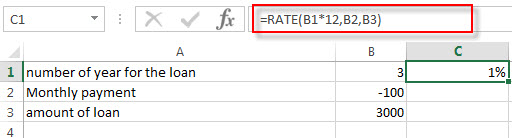# Excel RATE Function

This post will guide you how to use Excel RATE function with syntax and examples in Microsoft excel.

### Description

The Excel RATE function returns the interest rate per payment period of an annuity.

The RATE function is a build-in function in Microsoft Excel and it is categorized as a Financial Function.

The RATE function is available in Excel 2016, Excel 2013, Excel 2010, Excel 2007, Excel 2011 for Mac.

### Syntax

The syntax of the RATE function is as below:

`=RATE(nper, pmt,pv,[fv],[type],[guess])`

Where the RATE function arguments are:

• nPer -This is a required argument. The total number of payments periods in an annuity.
• Pmt – This is a required argument. The amount of the payment made each period.
• Pv – This is a required argument. The present value of the payments.
• Fv – This is an optional argument. The future value or a cash balance you want to attain after the last payment is made. If fv is omitted, it is assumed to be 0 (zero), that is, the future value of a loan is 0.
• Type – This is an optional argument. The number 0 (zero) or 1 and indicates when payments are due.
 Set type equal to If payments are due 0 or omitted At the end of the period 1 At the beginning of the period
• Guess – This is an optional argument. An initial guess at the internal rate of return. if it is omitted, and it will be set as 0.1 or 10%.

Note:

• Make sure that you are consistent about the units you use for specifying rate and nper. If you make monthly payments on a four-year loan at an annual interest rate of 12 percent, use 12%/12 for rate and 4*12 for nper. If you make annual payments on the same loan, use 12 percent for rate and 4 for nper.

### Excel RATE Function Examples

The below examples will show you how to use Excel RATE Function to calculate the interest rate per period of an annuity.

#1 to get the monthly rate of the loan, using the following formula:

`=RATE(B1*12,B2,B3)`### Related Functions

• Excel FV Function
The Excel FV function used to calculate the future value of an investment based on a constant interest rate. The syntax of the FV function is as below:=FV(rate,nper,pmt,[pv],[type])…
• Excel IPMT Function
The Excel IPMT function used to calculate the interest payment for an investment based on a constant payment schedule and a constant interest rage.The syntax of the IPMT function is as below:= IPMT (rate, per, nper, pv, [fv], [type])…
• Excel IRR Function
The Excel IRR function returns the internal rate of return for a series of cash flows and the cash flows must be occurred at regular intervals (monthly or annually).The syntax of the IRR function is as below:=IRR(values, [guess])…
• Excel ISPMT Function
The Excel ISPMT function used to calculate the interest paid during a specific period of an investment.The syntax of the ISPMT function is as below:= ISPMT (rate, per, nper, pv)…
• Excel MIRR Function
The Excel MIRR function returns the modified internal rate of return for a series of cash flows and the cash flows must be occurred at regular intervals (monthly or annually). The syntax of the MIRR function is as below:=MIRR(values, finance_rate,reinvest_rate)…
Excel NPER Function
The Excel NPER function returns the number of periods for an investment or loan based on periodic payment amount and a constant interest rate.The syntax of the NPER function is as below:= NPER (rate, pmt, pv, [fv], [type])…
• Excel NPV Function
The Excel NPV function returns the net present value of an investment by using a discount rate and a series of future cash flows (future payments and income).The syntax of the NPV function is as below:= NPV (rate, Value1,[value2],…)…
• Excel PMT Function
The Excel PMT function returns the payment amount for a loan or investment based on constant payments and a constant interest rate.The syntax of the PMT function is as below:= PMT(rate, nper, pv,[fv],[type])…
• Excel PPMT Function
The Excel PPMT function returns the payment amount on the principal for a given period for a loan or investment based on constant payments and a constant interest rate. The syntax of the PPMT function is as below:=PPMT(rate, per,nper, pv,[fv],[type])…
• Excel PV Function
The Excel PV function returns the present value of a loan or investment based on constant payments and a constant interest rate. So you can use the PV function to get the present value based on a series of future payments.The syntax of the PV function is as below:= PV(rate,nper,pmt,[fv],[type])…